# Digital Encoder or Binary Encoder

When we insert any character or symbol to a digital system, through key board, it is needed to be encoded in machine readable farm. Digital systems like computer etc, cannot read the characters or symbol directly. The system reads and computes any characters, numbers and symbols in their digital form. An encoder does the job that means, it converts different human readable characters or symbol to their equivalent digital format. An encoder is basically multi inputs and multi outputs digital logic circuit, which has as many inputs as the number of character to be encoded and as many outputs as the number of bits in encoded form of characters.

Suppose we have to design an encoder which will encode 10 characters (from 0 to 9). The encoded form of each character would be 4 bit binary equivalent. Then the encoder will have 10 numbers of input lines and each for one character. There will be four output lines to represent 4 bit encoded form of each input character.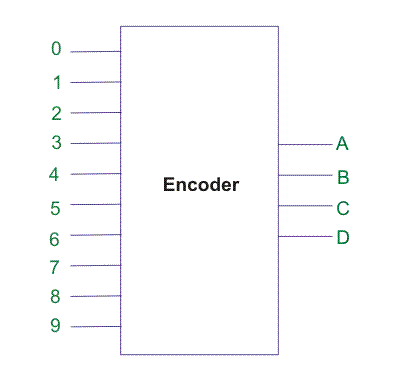Similarly for encoding M numbers of characters in N bit format, we need M input N output digital encoder.
In encoder normally, the input of which encoding to be done, is made high, other all inputs remain low at that time. That means a digital encoder works on active high input.
To understand about a digital encoder let us design the above decimal to binary encodes. The truth table for 10 inputs 4 output encoder would be,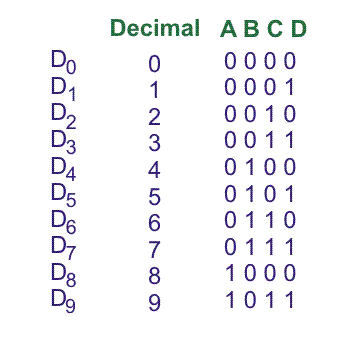From truth table it is found, that output A would be high at D8, D9.
So, it can be written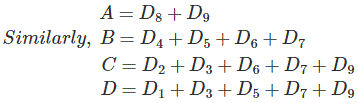From above 4 equations the logic circuit drawn as follows,
Figure:3
This circuit can also be considered as Decimal to BCD encoder.

## Octal to Binary Encoder

The octal numbers system has bas of 8. Hence the number of digits used in octal system is 8 and the octal digits are 0 to 7. Hence, there will be eight input line in a basic Octal to binary encoder. As binary equivalent of numbers 0 to 7 can be represented by only three binary bits, there will be three output lines to represent bits of binary equivalent of octal number.
The truth table logical relations between inputs and outputs and corresponding logic circuit are shown as follows,

 Octal Digit Binary Equivalent A B C D0 → 0 0 0 0 D1 → 1 0 0 1 D2 → 2 0 1 0 D3 → 3 0 1 1 D4 → 4 1 0 0 D5 → 5 1 0 1 D6 → 6 1 1 0 D7 → 7 1 1 1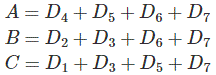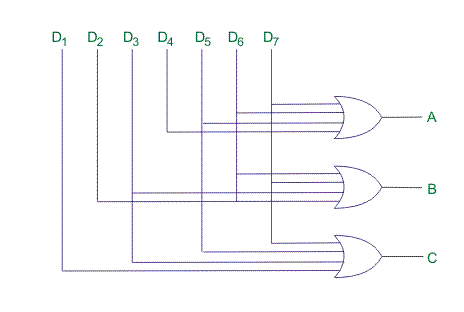Want To Learn Faster? 🎓
Get electrical articles delivered to your inbox every week.
No credit card required—it’s 100% free.